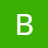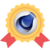# Creative Communities of the World Forums

The peer to peer support community for media production professionals.

Forums Adobe After Effects Audio levels triggered by markers

• # Audio levels triggered by markersBoston Cainfraupdated 1 week, 3 days ago
3 Members · 3 Posts
• ### Jean Livenais

March 10, 2023 at 5:59 am

Hello,

I have an expression which allows to control audio levels transitions triggered by markers with values in the comments.

It is working but when the playhead is before the first marker, it show an error.

Can you help me to adapt the expression so that the error doesn’t show?

n=0;

m=thisLayer.marker;

if (m.numKeys > 0){

n = m.nearestKey(time).index;

if (time < m.key(n).time) n–;

if (n > 0){

t = time – m.key(n).time;

if (m.key(n).value)

{

L1 = eval(m.key(n).comment.split(” “));//-50;

L2 = eval(m.key(n).comment.split(” “));//-12;

else{

}

}else{[L1,L1]}

}

[v,v]

• ### Dan EbbertsMarch 11, 2023 at 12:03 am

It looks like when n is 0 (before the first marker) L1 is not defined so this line will fail:

}else{[L1,L1]}

• ### Boston CainfraMarch 11, 2023 at 1:40 am

Hello Jean Livenais

NOTE- Not all error code is nothing

It might be pointing you to the way forward but if you can decode it , you can be pixee of or even fed up

Have finally decided and solved it for you with the meaning of each which will help you understand how to solve the errors

“n=0;

if (m.numKeys > 0){
n = m.nearestKey(time).index;
if (time < m.key(n).time) n–;
if (n > 0){
t = time – m.key(n).time;
if (m.key(n).value)
{

L1 = eval(m.key(n).comment.split(” “));//-50;
L2 = eval(m.key(n).comment.split(” “));//-12;
else{
}
}else{[L1,L1]}
[v,v]”

This code, which calculates a marker’s value based on its parameters at a particular time, was built in the scripting language of Adobe After Effects.

Two variables, n and m, are initially initialized in the code. Whereas m is given the value of the marker for the current layer, n is given the value of zero.

Then, it determines whether the marker contains any keyframes. If it does, it uses the nearestKey() method to identify the keyframe that is the closest to the current time (in seconds) and assigns its index to the variable n.Answer is
“n=0;
m=thisLayer.marker;

if (m.numKeys > 0){
n = m.nearestKey(time).index;
if (time < m.key(n).time) n–;
if (n > 0){
t = time – m.key(n).time;
if (m.key(n).value)
{

L1 = eval(m.key(n).comment.split(” “));//-50;
L2 = eval(m.key(n).comment.split(” “));//-12;
else{
}
}else{[L1,L1]}
[v,v]”

This code, which calculates a marker’s value based on its parameters at a particular time, was built in the scripting language of Adobe After Effects.

Two variables, n and m, are initially initialized in the code. Whereas m is given the value of the marker for the current layer, n is given the value of zero.

Then, it determines whether the marker contains any keyframes. If it does, it uses the nearestKey() method to identify the keyframe that is the closest to the current time (in seconds) and assigns its index to the variable n.

Thank you

Viewing 1 - 3 of 3 posts